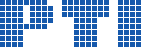Polish Information Processing Society# Genetic Algorithms for Balanced Spanning Tree Problem

## Riham Moharam, Ehab Morsy, Ismail Ismail

Citation: Proceedings of the 2015 Federated Conference on Computer Science and Information Systems, M. Ganzha, L. Maciaszek, M. Paprzycki (eds). ACSIS, Vol. 5, pages 537545 ()

Full text

Abstract. Given an undirected weighted graph G=(V,E) with vertex set V and edge set E and a designated vertex r in V, we consider the problem of constructing a spanning tree in G that balances both the minimum spanning tree and the shortest paths tree rooted at r. Formally, for any two constants A,B > =1, we consider the problem of computing an (A,B)-balanced spanning tree T in G, in the sense that, (i) for every vertex v in V, the distance between r and v in T is at most A times the shortest distance between the two vertices in G, and (ii) the total weight of T is at most B times that of the minimum tree weight in G. It is well known that, for any A, B > = 1, the problem of deciding whether G contains an (A,B)-balanced spanning tree is NP-complete. Consequently, given any A > = 1 (resp., B > = 1), the problem of finding an (A,B)-balanced spanning tree that minimizes B (resp., A) is NP-complete. In this paper, we present efficient genetic algorithms for these problems. Our experimental results show that the proposed algorithm returns high quality balanced spanning trees.